﻿ Divide
Liquid Studio 2021
Data Mapping / Data Mapper Functions / By Category / Maths / Divide
In This Topic
Divide
In This Topic

Divide
Maths
##### Icon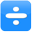##### Description
Divide one numeric value by another
##### Inputs
 Dividend Value to divide Divisor Value to divide by
##### Outputs
 Result Result of dividing first input by second
##### Properties
 None -

##### Usage

The Data Mapper Maths Divide function allows you to carry out division on input values. You can use the Divide function with input source data or in conjunction with other arithmetic functions in the Maths section. To apply the Maths Divide function, use the following process:

Create a new Data Mapper file, dragging your XML data source and targets into the editor area. For this example we are using payroll_source.xsd, an XML Schema Definition inferred from the following source:

Source XML
Copy Code
```<payroll>
<employee payroll_id="126">
<rate>9.45</rate>
<hours>35</hours>
<bonus>70.00</bonus>
<funding>350</funding>
</employee>
<employee payroll_id="129">
<rate>10.50</rate>
<hours>30</hours>
<bonus>150.00</bonus>
<funding>500</funding>
</employee>
<employee payroll_id="130">
<rate>8.90</rate>
<hours>40</hours>
<bonus>100.00</bonus>
<funding>550</funding>
</employee>
<employee payroll_id="133">
<rate>11.20</rate>
<hours>40</hours>
<bonus>200.00</bonus>
<funding>600</funding>
</employee>
</payroll>
```

For our target schema we will be using payroll_target.xsd, inferred from the following XML:

Target XML
Copy Code
```<wages>
<payee>
<ref>122</ref>
<result type="hourly">7.90</result>
</payee>
<payee>
<ref>125</ref>
<result type="hourly">8.45</result>
</payee>
<payee>
<ref>126</ref>
<result type="hourly">9.10</result>
</payee>
</wages>
```

The data models payroll data for temporary staff within an organisation. The output is designed to capture some value calculated from the source, using the "type" attribute to indicate what the calculation result represents. Let's assume that we want to calculate the actual hourly rate for each employee, including their bonus. We will need to carry out an addition calculation in conjunction with the division. Here is the Data Mapper with Source and Target imported:Drag the Maths Divide function from the Component Palette into the mapping area: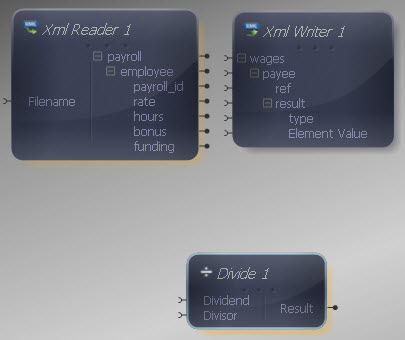The Divide component has two inputs, representing the value to divide and the value to divide it with. Both inputs and output of the Divide function may be connected to items in the XML Reader/ Writer or other components in the Mapper.

Connect the Dividend input in the Divide component to the "bonus" output in the XML Reader. Then connect the Divisor input to the "hours" output, so that the result will be the bonus amount per hour.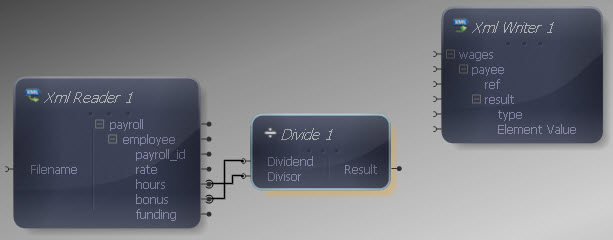Now we need to add the division result to the basic hourly rate for each employee. Drag an Add component onto the Mapper, connecting its inputs to the "rate" output in the XML Reader and the output of the Divide function.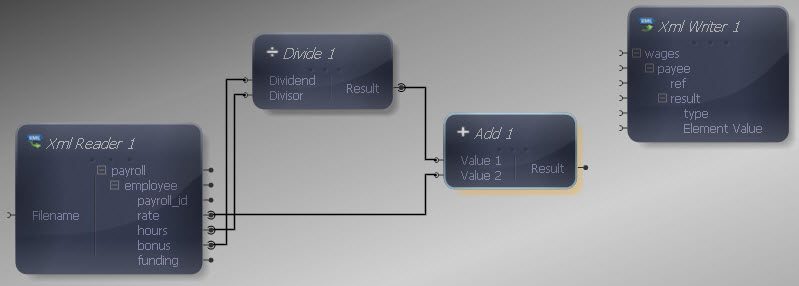Now connect the Add output to the Element Value input in the XML Writer, so that the addition result is written into the "result" element.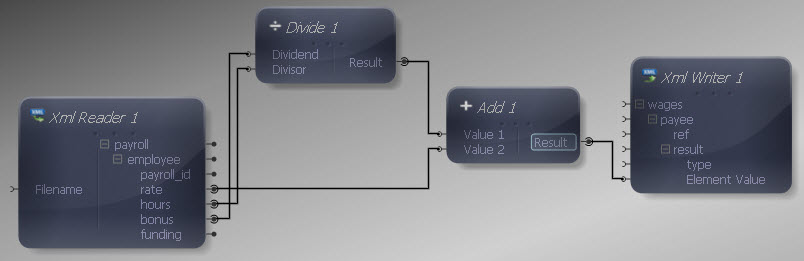Now we need to include the "type" attribute in the source, so add a Constant Value from the Data Type section. Right-click it and choose Show Properties. Choose a String Data Type and enter "hourly" as the value.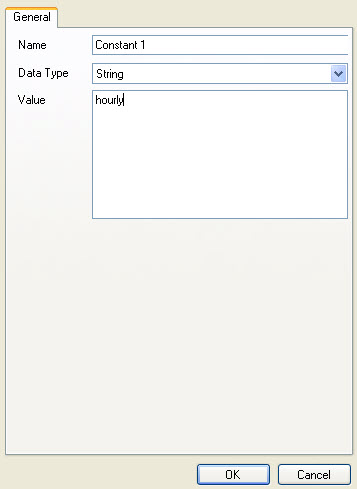Connect the Constant output to the "type" input in the XML Writer.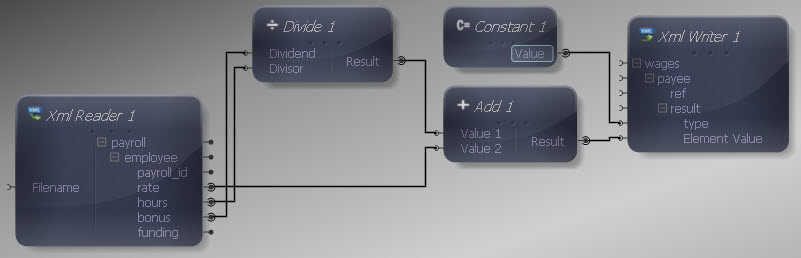Finally let's make the remaining input and output connections. We do not want to map all data items in the source, as we are only interested in certain values. Map "payroll" to "wages", "employee" to "payee" and "payroll_id" to "ref". We don't need to map anything to the "result" element as it will be written out each time the Mapper maps the addition result to its Element Value. Although we have used some of the remaining input values for calculations, we do not need to map them individually to the target, as we are mainly interested in the calculation result.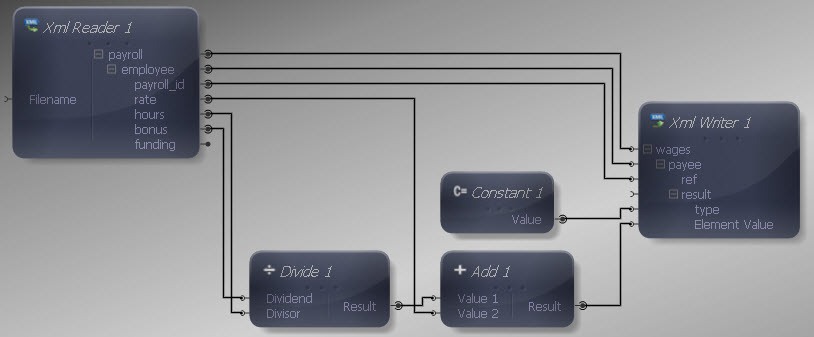We can now execute the transform by pressing Shift-F5 or the Execute button (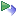). The transform is applied and the file we selected as output opens in the editor:

Output XML
Copy Code
```<wages>
<payee>
<ref>126</ref>
<result type="hourly">11.45</result>
</payee>
<payee>
<ref>129</ref>
<result type="hourly">15.50</result>
</payee>
<payee>
<ref>130</ref>
<result type="hourly">11.40</result>
</payee>
<payee>
<ref>133</ref>
<result type="hourly">16.20</result>
</payee>
</wages>
```

The output contains an indicator of the actual hourly rate paid to each employee.

This is a scalar function so the standard rules apply if multiple values are presented to one or more inputs.